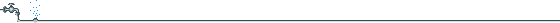###MCL CalculationWhat is equivalent daily human intake for an average human?

Intake = RfD × body weight = 1 × 70 = 70 mg/day
(Assume RfD = 1 mg/kg/day)

What concentration in drinking water would provide this intake?

Water concentration = intake/water consumption

= 70mg/day divided by 2 liter/day

= 35 mg/liter

But water consumption is assumed to be only 20% of total consumption,

MCL = 35 mg/liter × 0.2 = 7 mg/liter

If chemical is Class C carcinogen, divide by ten, so in this case:

MCL = 7/10 = 0.7 mg/liter

If chemical is Class A or B carcinogen, then:

MCL = Practical Quantitation Limit